# Linear Methods and Autoencoders in Recommender SystemsSep 7

Linear regression is probably the simplest and surprisingly efficient machine learning method. It should be the method of your first choice, according to the famous KISS principle. Also, it often works better than sophisticated methods, because it is quite difficult to overfit training data with linear models, thus their generalization performance is not hurt by overfitting.

On the other hand, linear models are weak learners, meaning that they tend to underfit data, because of their limited capacity to capture complex relationships. Combining multiple linear models doesn’t help much either, because it is hard to enforce diversity among them (even with negative correlation learning) and the resulting ensemble is often close to a linear model again.

Nevertheless, for sparse multidimensional input data, linear models have always been a good match, and hard to beat when used as a baseline. And in recommender systems, almost every dataset of user-item ratings is sparse and multidimensional.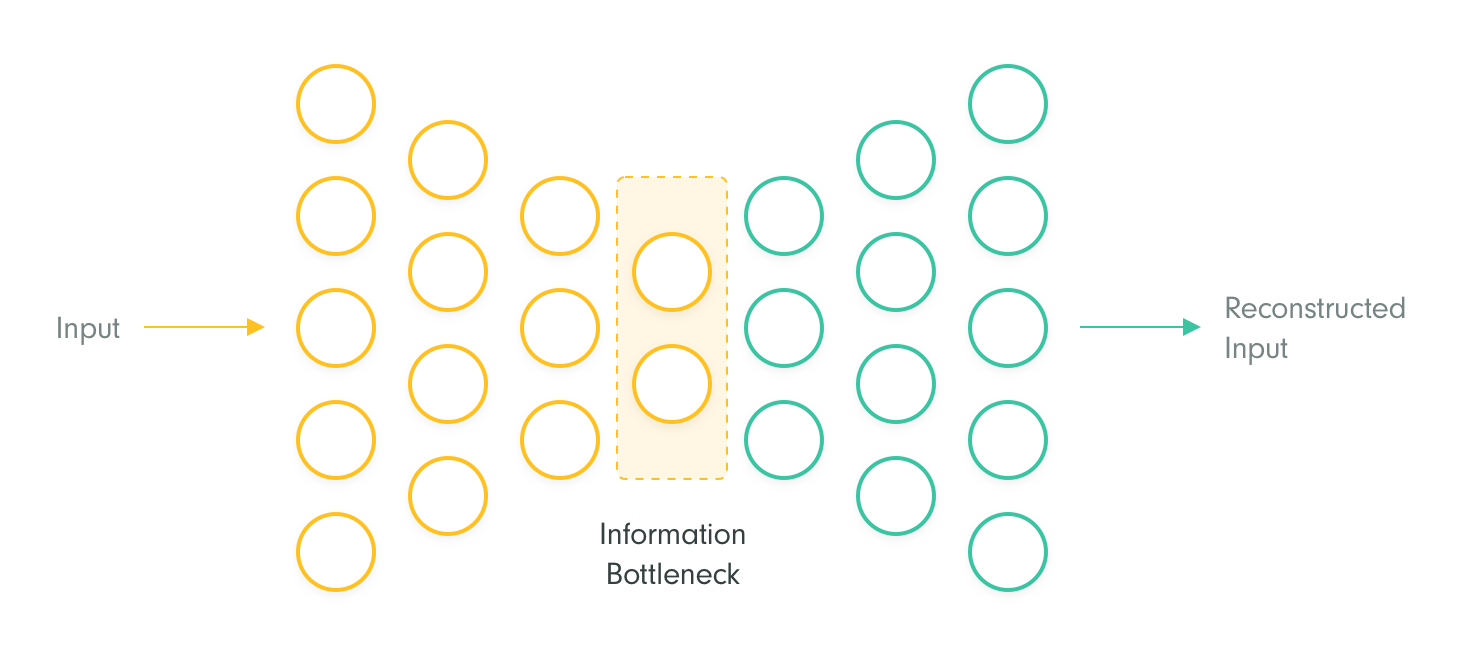Autoencoder is a special model trained to reconstruct input vectors, meaning that input and output vectors should match, despite the information bottleneck in the hidden layer. You train autoencoders to minimize this reconstruction error on unseen data vectors. The information bottleneck is implemented for example by reducing and subsequently increasing dimensionality of input vectors, by regularizing models to enforce sparsity in the hidden layer, or by a combination of such methods. In computer vision, autoencoders are successfully used to reconstruct images, where minimizing the reconstruction error can be further extended by employing a perceptual loss.

Deep autoencoders are multilayered networks reducing the dimensionality of images (encoder) and subsequently increasing the dimensionality again (decoder). Those are typically realized by convolutional networks, because images have special properties (e.g. color of pixels in the neighborhood is strongly correlated). Such architectures are also utilized in recommender systems when producing compressed feature vectors (neural embeddings) of items to be recommended from their images.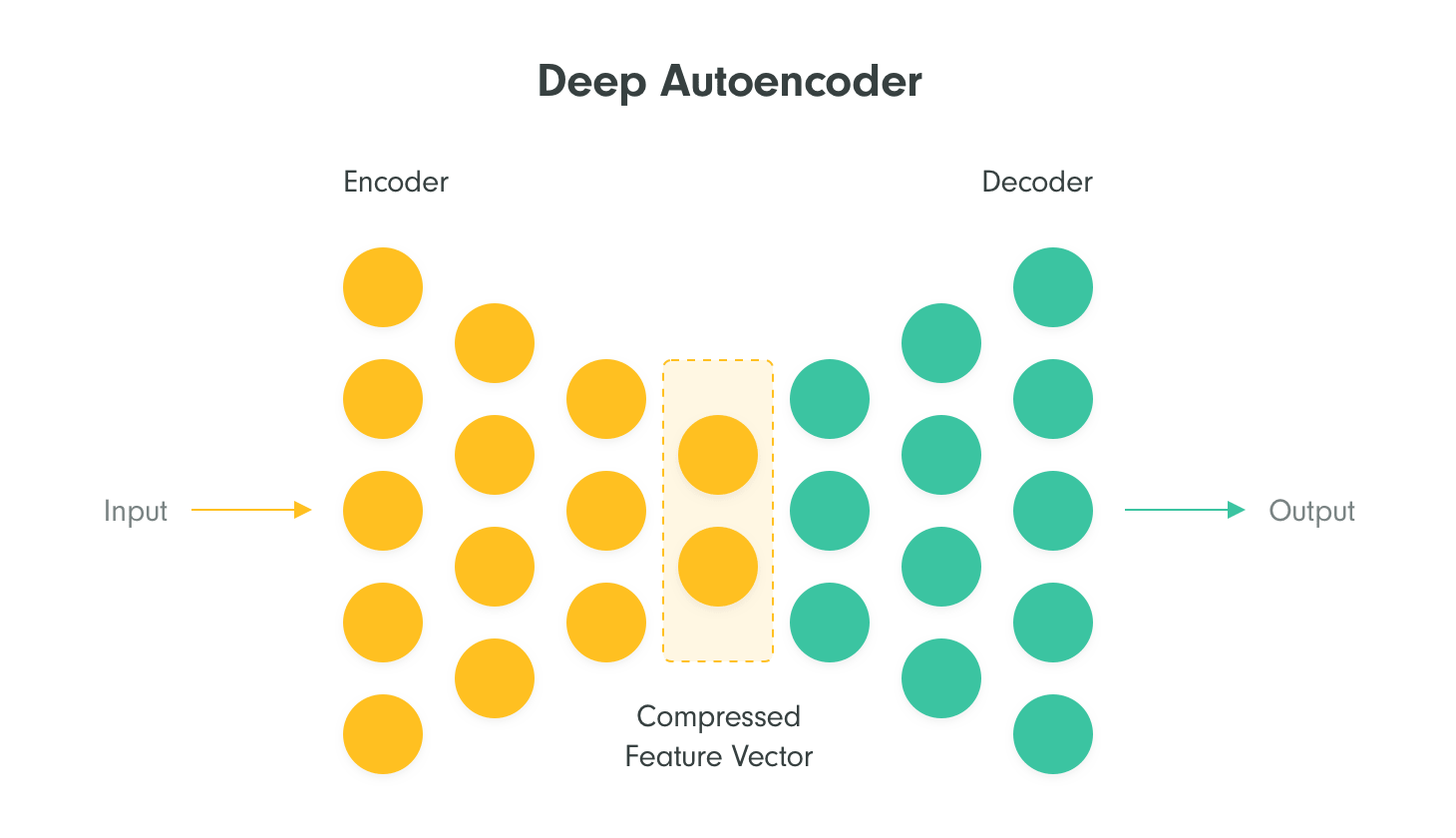The output of a deep autoencoder should match the input as well as possible. To minimize the reconstruction error, various training techniques and architectures have been developed.

The user-item matrix commonly used in Recommender systems (called the rating or implicit preference matrix) is significantly different from images. The absence of local similarities and autocorrelations in sparse multidimensional rating matrices makes utilizing autoencoders from computer vision difficult.

## Linear Autoencoders in Recommender Systems

In the case of recommender systems, you can predict the rating of an item using linear models from the rating (or implicit feedback) matrix. Sparse Linear Method (SLIM) trains a sparse weight matrix with a zero diagonal to “reconstruct” the original matrix. Meaning that a recommendation score of an item for a given user is computed as a weighted linear combination of other items that have been rated by the user. The size of the square weight matrix matches the number of items. Therefore it is a special case of sparse matrix factorization. SLIM can also be classified as a sparse autoencoder. The encoding part is an identity (no compression of the user vectors) and decoder is the weight matrix transpose. The only information bottleneck here is the zero diagonal of the weight matrix.

In case there can be 1 in the diagonal of the weight matrix, any machine learning algorithm would quickly discover a trivial solution to reconstruction of the rating matrix - place 1 on the diagonal and 0 elsewhere. When diagonal weights are forced to be zero, the machine learning algorithm has to reconstruct the rating using similar items.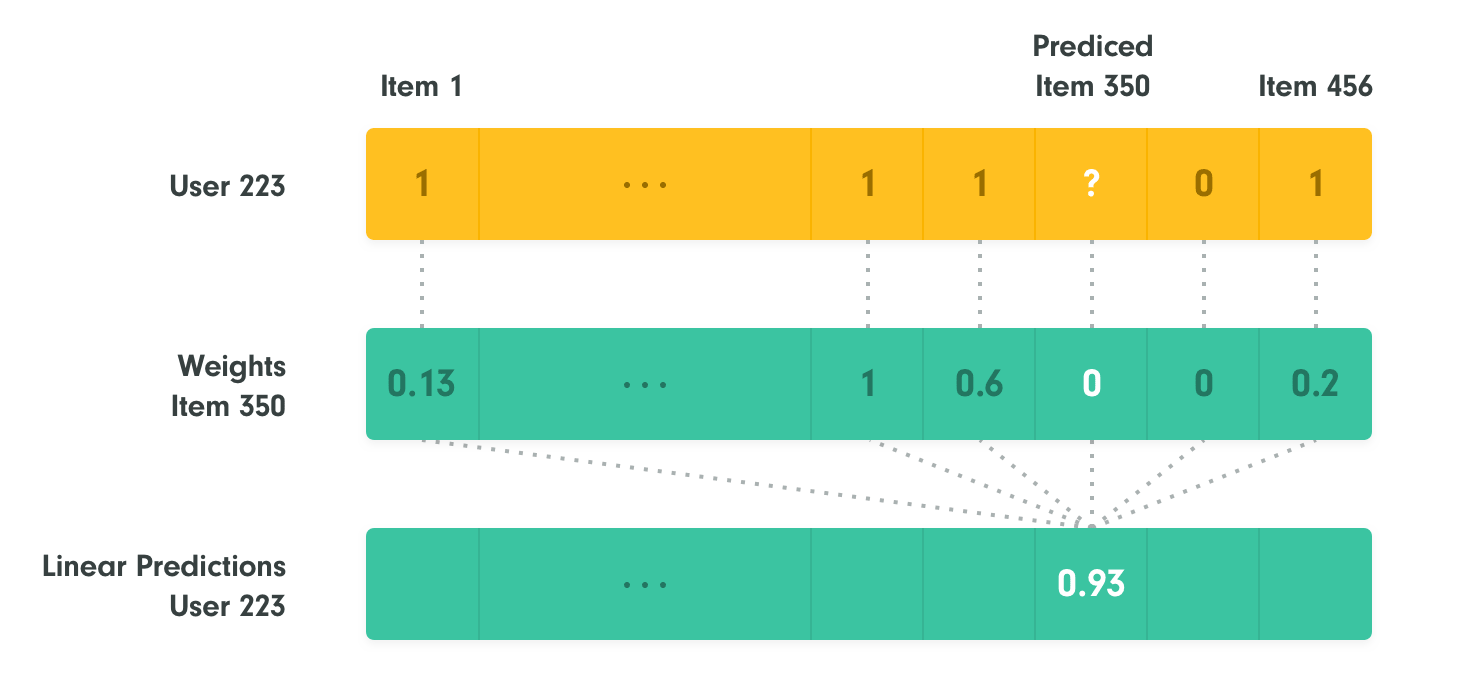Here is an example of how the other items that have been rated by a particular user (223) are summed up weighted by their similarities (or co-occurrence) with predicted item (350).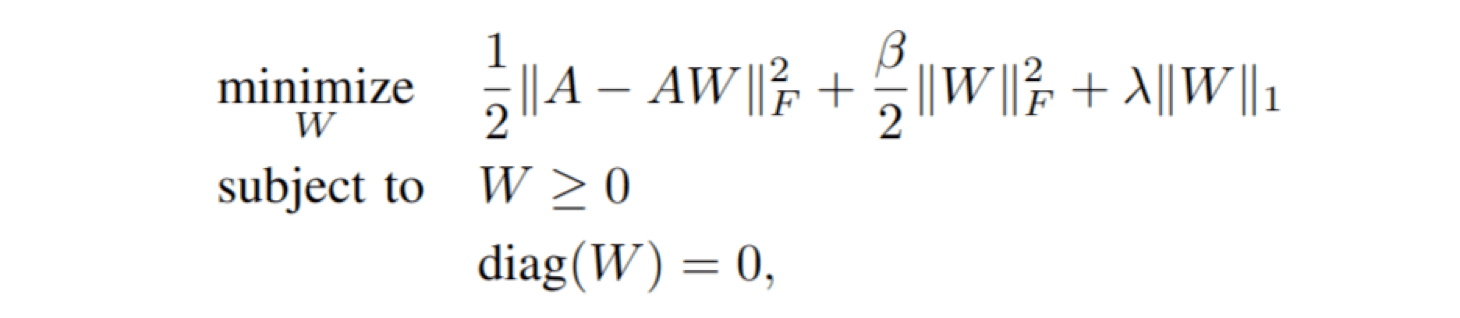SLIM training is straightforward. Reducing the squared reconstruction error (of the Rating matrix A) while regularizing the weight matrix (W) to enforce sparsity and keeping the diagonal zero to avoid a trivial solution. It has been shown later that the stochastic gradient descent works better than the optimization technique proposed in the original SLIM paper.

The trained sparse weight matrix can be also used to generate explanations. This item is recommended, because the user rated items that obtained the highest weights in the column of the sparse weight matrix and are therefore considered most similar to the recommended item.

Embarrassingly Shallow Autoencoders for Sparse Data (EASE) from Harald Steck are quite similar to SLIM, but the weight matrix is dense and training is done in one step by generalized least squares optimization using the matrix inversion. Both SLIM and EASE have difficulties with datasets where a number of items is large, because of the size of the weight matrix. For more details, have a look at the presentation of Harald Steck on linear models in recommendation.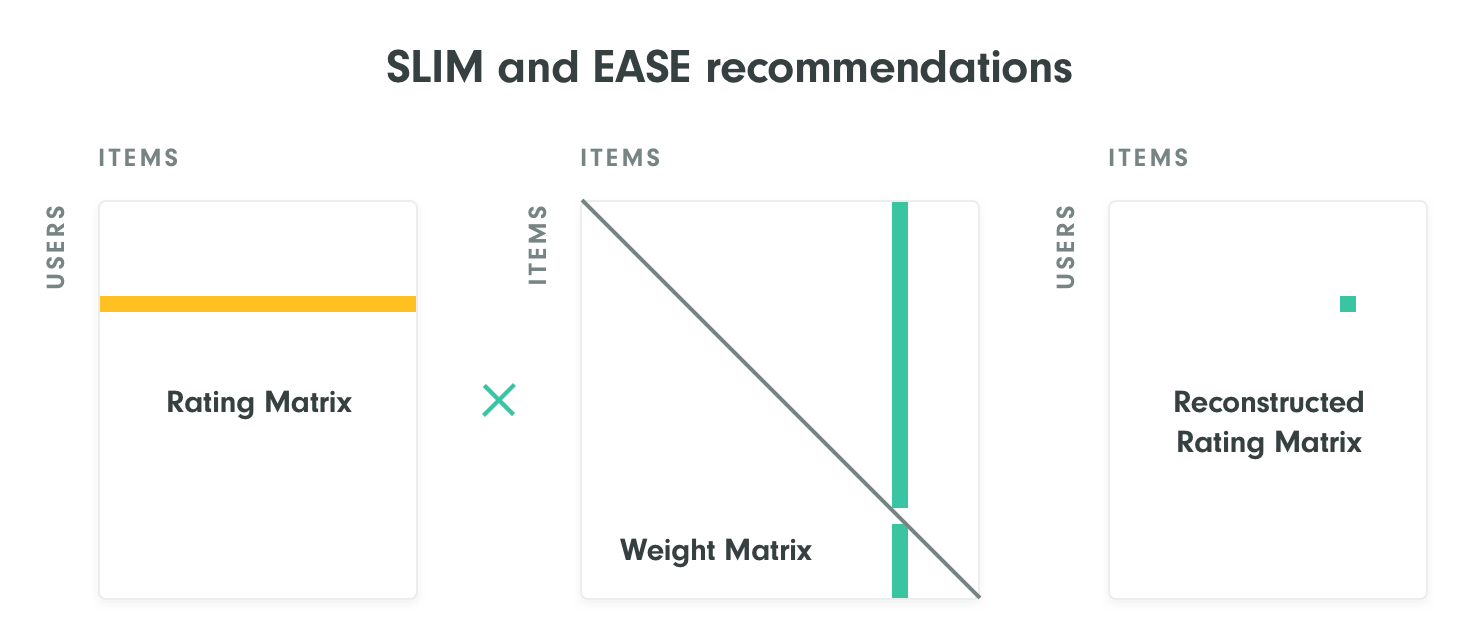The reconstruction of the rating matrix is intuitive - for each item, look at all other items the user has rated (except the predicted item) and increase/decrease the predicted rating based on the corresponding weights (similarity/dissimilarity to predicted item). While SLIM allows only non-negative weights in the Items-to-items matrix, EASE shows that allowing negative weights (dissimilarities) is beneficial.

Note that it is also possible to use a low rank approximation of the rating matrix instead of the sparse items-to-items weight matrix. This solves the scalability issues, because the number of latent features can be as low as a couple of hundreds even for massive datasets. These linear methods (matrix factorization or singular value decomposition) are quite popular in recommender systems. Again, these methods are linear autoencoders and we use their codes (latent embeddings) extensively in Recombee.

The lower is the dimensionality (k) of latent codes, the bigger is the information bottleneck. User vector (row of a rating matrix) is encoded and compressed into a user embedding, item vector (column) is encoded into a latent vector in the item latent matrix. Decoding involves multiplying corresponding user and item latent vectors for each element of the reconstructed rating matrix. Because the reconstruction is a linear operation, the encoder should be also linear even when latent matrices are trained by sophisticated optimization algorithms. For more details, check out this great doctoral thesis on low rank approximation methods for matrix factorization including handling cold start vectors.

## Non-linear Autoencoders

Shallow linear autoencoders are weak learners. The nonlinear relations can be modelled by more sophisticated autoencoders, for example variational autoencoders with fully connected layers.

While the code (embedding) of linear autoencoders is just a linear projection of the rating matrix (similar to PCA, but minimizing the reconstruction error does not necessary lead to orthogonal latent features), non-linear autoencoders use multiple layers of neurons with e.g. sigmoid activation functions to compress and decompress the rating matrix. The size of the encoder and decoder does not need to grow proportionally with the size of the rating matrix, therefore their scalability is better than in case of EASE or SLIM models.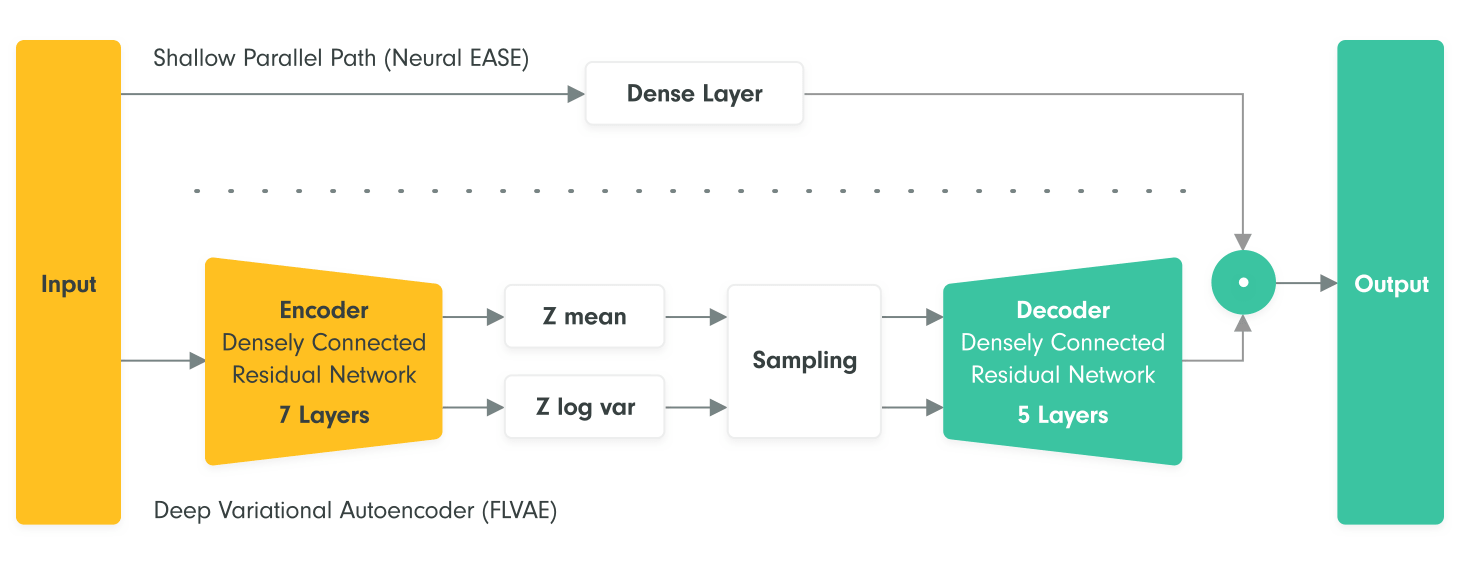In the next post, we will introduce our VASP recommendation algorithm, taking the advantage from both linear and non-linear autoencoders. VASP is currently a state of the art algorithm on the popular Movielens 20M dataset.

Recommendation Engine